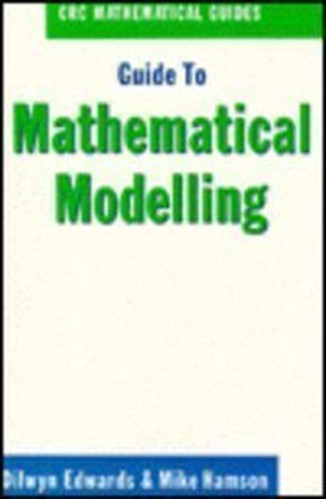# Guide to Mathematical Modelling by Dilwyn Edwards (Basingstoke, England)

In Stock
£28.99
An introduction to mathematical modelling. It explains the concept of a mathematical model, examines why mathematical modelling is so important, and presents several different modelling situations, ranging from simple to very complex.
Only 1 left

## Guide to Mathematical Modelling Summary

### Guide to Mathematical Modelling by Dilwyn Edwards (Basingstoke, England)

Guide to Mathematical Modelling is a book designed for students and professionals interested in an introduction to mathematical modelling. It explains the concept of a mathematical model, examines why mathematical modelling is important, and presents several different modelling situations, ranging from simple to very complex. The book also discusses communication and the art of presenting and reporting on modelling activities.

### Why buy from World of BooksOur excellent value books literally don't cost the earthFree delivery in the UKEvery used book bought is one saved from landfill

WHAT IS MODELLING? Introduction. Models and Modelling. The Learning Process for Mathematics Modelling. Summary. GETTING STARTED. Introduction. Examples. Conclusion. Further Examples. Further Comments on the Examples in Section 2.2. MODELLING METHODOLOGY. Introduction. Definition and Terminology. Methodology and Modelling Flow Chart. The Methodology in Practice. Summary. UNITS and DIMENSIONS. Introduction. Units. Dimensions. Dimensional Analysis. Summary. MODELLING SKILLS. Introduction. Listing Factors. Making Assumptions. Types of Behaviour. Translating into Mathematics. Choosing Mathematical Functions. Relative Sizes of Terms. Reducing the Number of Parameters. Summary. USING DATA. Introduction. Data Collection. Empirical Models. Estimating Parameters. Errors and Accuracy. Testing Models. Summary. USING RANDOM NUMBERS. Introduction. Modelling Random Variables. Generating Random Numbers. Simulations. Using Simulation Models. Packages and Simulation Languages. Summary. USING DIFFERENTIAL EQUATIONS. Introduction. First Order, One Variable. Second Order, One Variable. Second Order, Two Variables (Uncoupled). Simultaneous Coupled Differential Equations. Summary. REPORT WRITING and PRESENTATIONS. Introduction. Report Writing. A Specimen Report. Presentation. EXAMPLE MODELS. Introduction. Doing the Dishes. Shopping Trips. Disk Pressing. Gutter. Turf. Parachute Jump, On the Buses. Further Battles. Snooker. Further Models. BIBLIOGRAPHY. SOLUTION to EXERCISES. INDEX.

GOR009938854
Guide to Mathematical Modelling by Dilwyn Edwards (Basingstoke, England)
Dilwyn Edwards (Basingstoke, England)